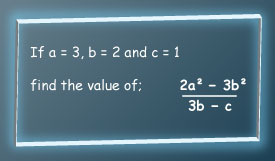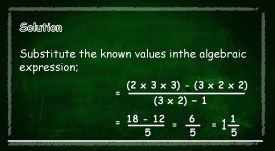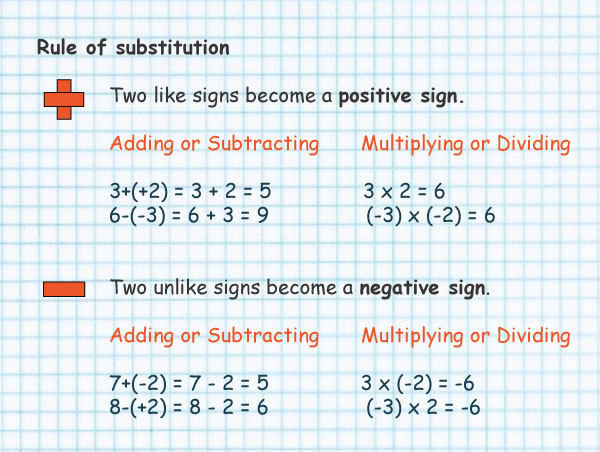Substitution in algebra

## Working out algebraic expression using substitution method

The word "Substitute" means to “replace” or “put in the place of another”.

When we work out the value of algebraic expressions using substitution we replace letters with numbers.

#### For example

if the expression given is; 2x-6 where x=10

Then we replace x with 10 in the expression; 2(10)-6=20-6=14

Another example

If x=4 then what is 24-5x

Solution; substitute x with 4 in 24-5(4) =24-20=4#### Example of how to work out the value of algebraic expressions using substitution### Substitution of negative numbers

When substituting negative numbers, put () around the numbers to get the calculations right.

Here is the rule of substitution to guide you.